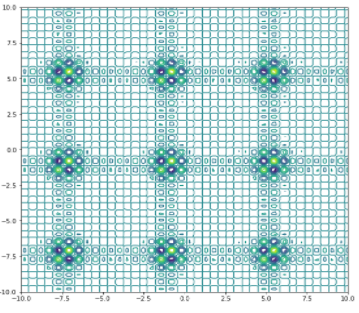top of page
Search

# Shubert Function

Updated: Jul 18, 2021Mathematical DefinitionInput Domain

It can define into any input domain but usually its evaluated on the square 𝑥𝑖 ∈ [−10,10] for all i= 1,2

Global Minima

It has 18 global minima 𝑓(𝑥 ∗ ) ≈ −186.7309.

Description and Features

Shubert function is continuous function.

The function is differentiable.

The function is non-separable.

The function is defined on n – dimensional space.

Python Implementation

```% Please forward any comments or bug reports in chat
Copyright 2021. INDUSMIC PRIVATE LIMITED. THERE IS NO WARRANTY, EXPRESSOR IMPLIED. WE DO NOT ASSUME ANY LIABILITY FOR THE USE OF THIS PROGRAM. If software is modified to produce derivative works, such modified software should be clearly marked. Additionally, user can redistribute it and/or modify it under the terms of the GNU General Public License. This program is distributed in the hope that it will be useful, but WITHOUT ANY WARRANTY. See the GNU General Public License for more details.
% for any support connect with us on help.indusmic@gmail.com
% Author: Ekta Kumari

import matplotlib.pyplot as plt
import numpy as np
from numpy import sin
from numpy import *
from numpy import pi
from numpy import sqrt
from matplotlib import cm

def f(x1,x2):
sum1=0
sum2=0
for i in range(1,6):
sum1 = sum1 + (i* cos(((i+1)*x1) +i))
sum2 = sum2 + (i* cos(((i+1)*x2) +i))
return sum1 * sum2

x1 =np.linspace(-10,10,100)
x2 =np.linspace(-10,10,100)
r_min,r_max= -10,10

x1,x2=np.meshgrid(x1,x2)
results=f(x1,x2)

figure=plt.figure(figsize=(9,9))
axis=figure.gca(projection='3d')
axis.contour3D(x1, x2, results,15)
axis.set_title('Shubert function')
axis.plot_surface(x1,x2,results, cmap=cm.rainbow)

axis.view_init(elev=21,azim=42)
axis.set_xlabel('X')
axis.set_ylabel('Y')
axis.set_zlabel('Z')
plt.contour(x1, x2, results,15)
plt.show()

```

References:

 Jamil, Momin, and Xin-She Yang. "A literature survey of benchmark functions for global optimization problems." International Journal of Mathematical Modelling and Numerical Optimization 4.2 (2013): 150-194.